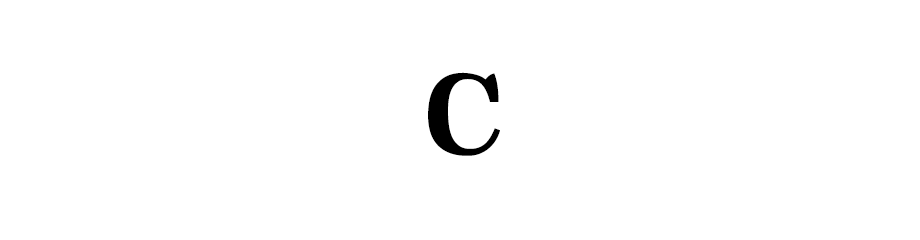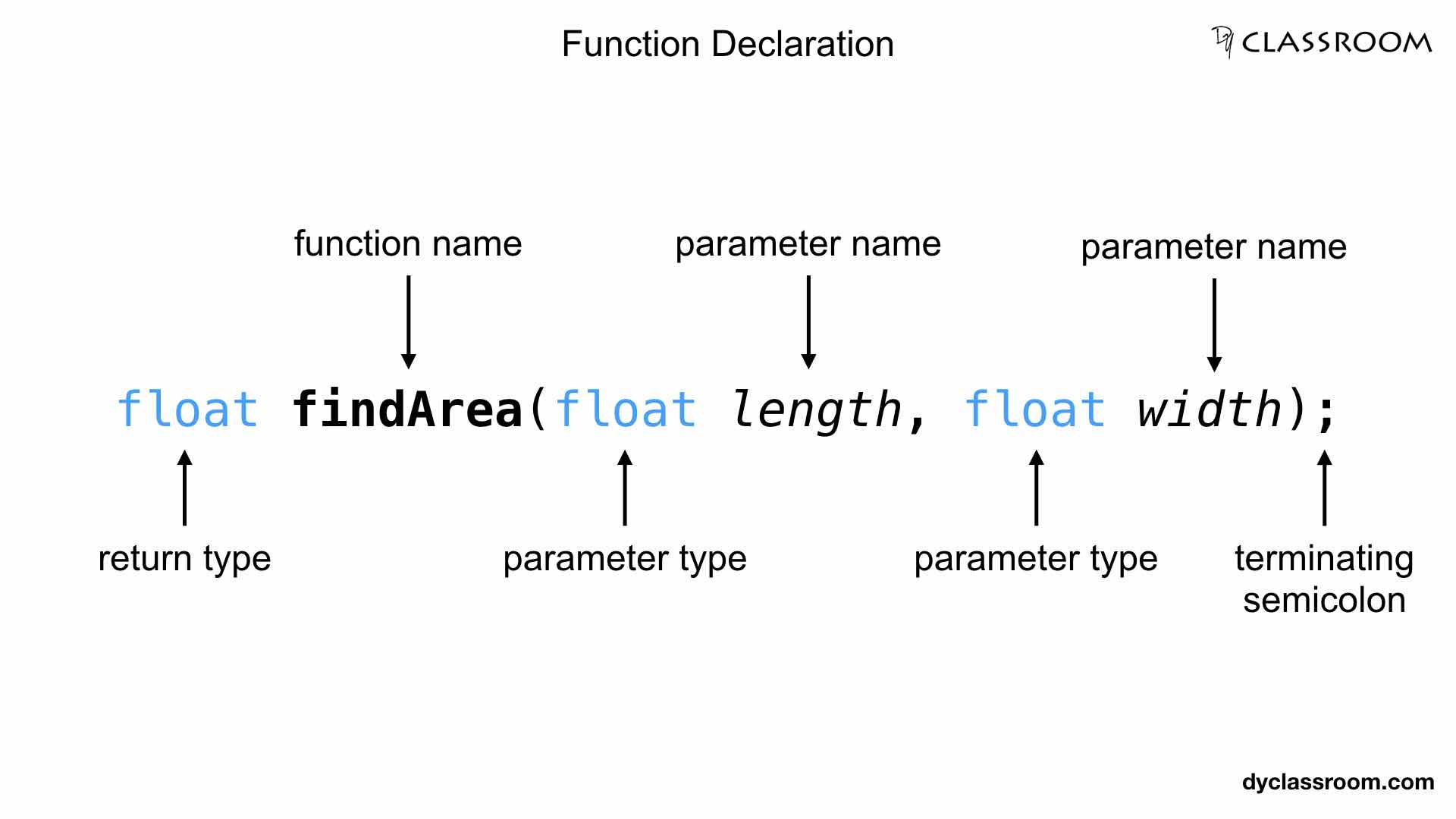# C - Functions

C ProgrammingIn this tutorial we will learn about functions in C programming language.

## What is a function?

A function in C is a block of code consisting of some statements that performs a specific task.

For example, we can have a `greetings()` function that will print greetings message whenever it is called.

## Types of functions

There are two types of functions in C.

• Library Functions
• User Defined Functions

### Library Functions

These are the functions that we get from the C library like `strlen()`, `concat()` etc.

### User Defined Functions

These are the functions created by the user like for example `greetings()` or `displayResult()` etc.

The `main()` function is user defined function whereas, `printf()` and `scanf()` are library functions.

### Difference between Library and User Defined functions

Library functions are already created and ready for use and we don't have to write it. Whereas, User defined functions are the ones that we have to write in order to use it.

## Why we need user defined functions?

User defined functions helps us to break our program into subprograms. These subprogram are designed to perform specific task and hence it makes the code easier to develop, test and debug.

The idea is to break the program into modules (subprogram) and develop the program in small pieces and then join them together to solve the problem.

For example, if we have a program to find the area of a rectangle then we can either write the entire code inside the `main()` function. Or we can divide the program into subprograms using function like `findArea()`. This will help us in developing the code easily and will also help in testing and debugging in case of any error.

## Three parts of a user defined function

A user defined function consists of three parts.

• Function Declaration
• Function Definition
• Function Call

## Function Declaration

Like variables, in C we have to first declare a function before calling it.

Function declaration is also known as the function prototype and it consists of 4 parts given below.

• Return type
• Name of the funtion
• List of parameters
• Terminating semicolon

In the following example we are declaring a function named `findArea` having return type `float` and it takes two parameters `length` and `width` of type `float`.

``````float findArea(float length, float width);
``````### Return type

Return type tells us about the type of value returned by the function.

If a function is going to return lets say an integer value then the return type will be set to `int`.

We set the return type to `void` if the function returns nothing.

### Function name

Name of the function can be any valid identifier like `display()`, `result()` etc.

Don't use reserved keywords to name a function.

### Parameter list

Parameter list must be separated by comma.

If a function takes no parameter then set it to `void`.

In the following example the `greetings()` function takes no parameter and returns nothing.

``````void greetings(void);
``````

Parameter names are optional and can be ignored in the function declaration.

In the following example we are not writing the parameter names.

``````float findArea(float, float);
``````

Parameter name in the function declaration and in function definition do not need to be the same.

### Terminating semicolon

The terminating semicolon `;` marks the end of the function declaration.

## Function Definition

This is the part were we implement the function. Function definition is also known as function implementation.

Function definition consists of 6 parts, three under Function Header and three under Function Body.

• Return type
• Function name
• List of parameters
• Function Body
• Declaration of local variables
• Function statements
• Return statement

The return type in function definition must match the return type in function declaration. And we already know that the return type tells us about the type of value returned by the function.

The function name in the function definition is same as the function name in the function declaration part.

The list of parameters in the function definition consists of the actual parameter names and do not have to match the parameter names in the function declaration.

### Function Body

This is the body of the function. We can declare local variables inside the body which is only accessible inside the function.

Then we can have other function statements to perform some task inside the function.

Finally we can have the return statement if the function returns any value. So, the return statement is optional.

In the following example we are implementing the `findArea()` function which we declared earlier in this tutorial.

``````float findArea(float length, float width) {
//variable declaration
float area;

//function statement
area = length * width;

//return statement
return area;
}
``````

## Function Call

This is the part were we call or invoke the function.

To call a function we write the name of the function and parenthesis and pass parameter list if the function needs any.

In the following example we are calling the `findArea` function and passing two values.

``````findArea(10, 20);
``````

## Write a program in C to find the area of a rectangle

In the following code we are breaking the program into subprograms using functions.

``````/**
* file: rectangle-area.c
* author: yusuf shakeel
* date: 2011-11-02
* description: rectangle-area program
*/

#include <stdio.h>

//function declaration
float findArea(float length, float width);

int main(void)
{
//variable
float length, width, area;

//input
printf("Enter length: ");
scanf("%f", &length);
printf("Enter width: ");
scanf("%f", &width);

//function call
area = findArea(length, width);

//output
printf("Area: %f\n", area);

printf("End of code\n");
return 0;
}

//function definition
float findArea(float length, float width) {
//local variable
float area;

//function statement
area = length * width;

//return statement
return area;
}
``````

Output

``````Enter length: 10
Enter width: 20
Area: 200.000000
End of code
``````